Function Repository Resource:

# ConfusionMatrixTrajectoryFunction

Construct a function that, when given a threshold probability value, produces a confusion matrix

Contributed by: Seth J. Chandler
 ResourceFunction["ConfusionMatrixTrajectoryFunction"][cmo,class] takes a ClassifierMeasurementsObject cmo and class, a designation of the positive class, and produces a function that, when applied to a threshold probability value, will generate a confusion matrix. ResourceFunction["ConfusionMatrixTrajectoryFunction"][assoc,class] takes an Association assoc derived from the application of a classifier to data.

## Details and Options

The output from ResourceFunction["ConfusionMatrixTrajectoryFunction"] will be a Function whose body has a Head of Through; the functions that Through will apply will consist of right compositions of a NearestFunction and two other functions.
The main purpose of this function is speed: it determines the trajectory of confusion matrices and associated statistics (such as false positive rate and true positive rate) without having to call ClassifierMeasurements repeatedly with varying UtilityFunction options.
Consistent with the way that ClassifierMeasurements works in creating receiver operating curves when there are more than two output classes, the confusion matrices produced by the function are one versus all. Even if originally the classifier was capable of outputting a large number of classes, all classes except the designated positive class are collapsed into a single class. Without this collapse, the receiver operating curve would become a receiver operating manifold in potentially many dimensions and would be difficult to visualize.
There is a correspondence between the threshold value and the disutilities placed on false positive and false negative responses from the classifier. A threshold of t corresponds to a relationship between false positive disutilities (fpc) and false negative disutilities (fnc) such that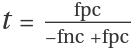.
In ResourceFunction["ConfusionMatrixTrajectoryFunction"][assoc,class], the Association assoc should have the keys "LogProbabilities", "TestSet" and "ExtendedClasses". See the example in the Scope section for a typical use case.

## Examples

### Basic Examples (2)

Get some simple training and test data:

 In:=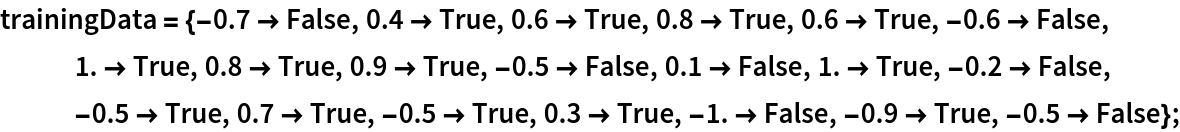In:=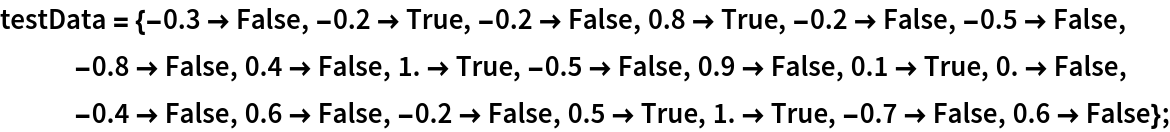Run a classifier and create a ClassifierMeasurementsObject:

 In:=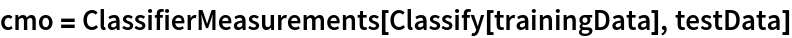Out=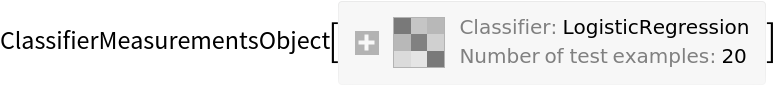Create the ConfusionMatrixTrajectoryFunction:

 In:=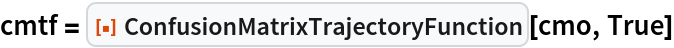Out=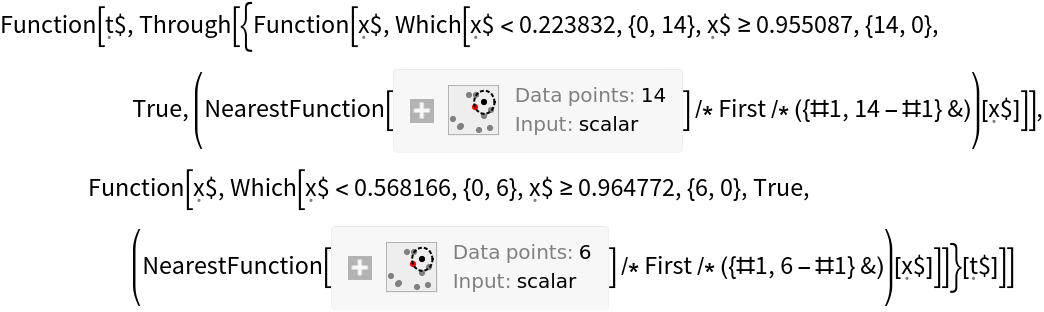Get the training and test sets of the Fisher iris data:

 In:=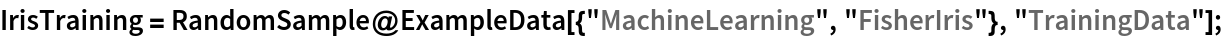In:=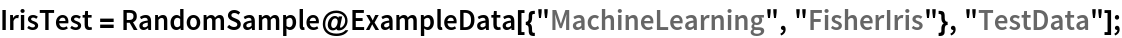Create a classifier on the training set:

 In:=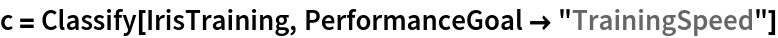Out=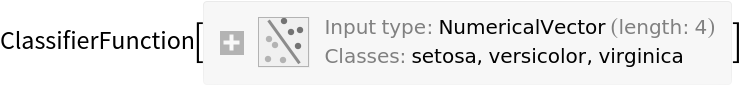Construct a ClassifierMeasurementsObject from the classifier and the test set:

 In:=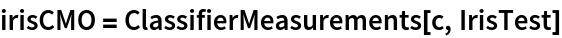Out=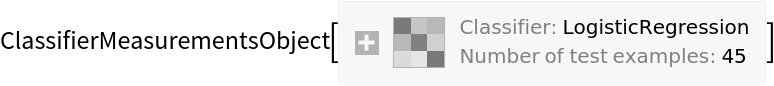Construct a function that will, for any given probability threshold, construct the confusion matrix when "setosa" is designated as the positive class:

 In:=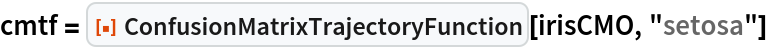Out=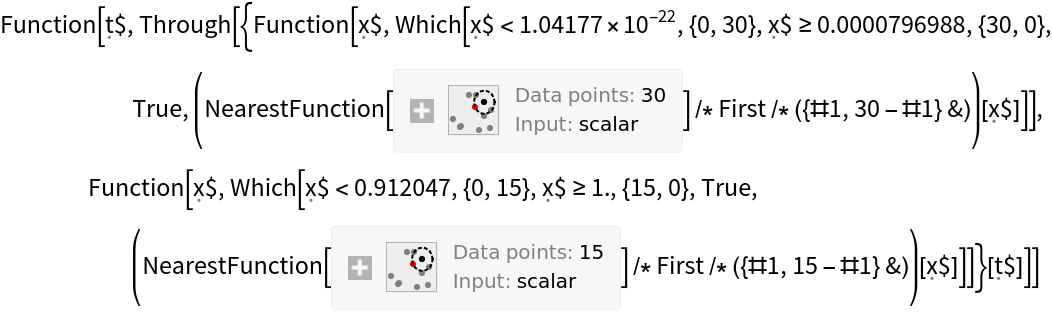Apply that function to a threshold of 0.62 to get a confusion matrix:

 In:=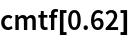Out=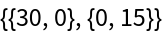Create a plot showing a trajectory of the false positive rate and true positive rate of the classifier for different thresholds:

 In:=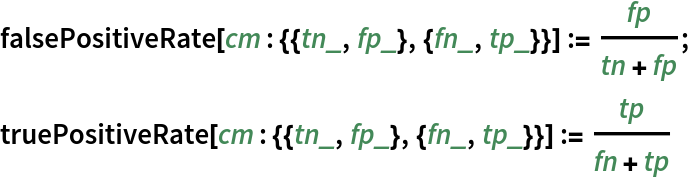In:=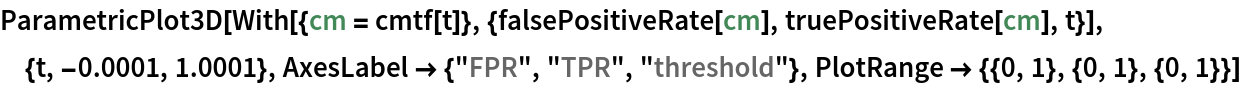Out=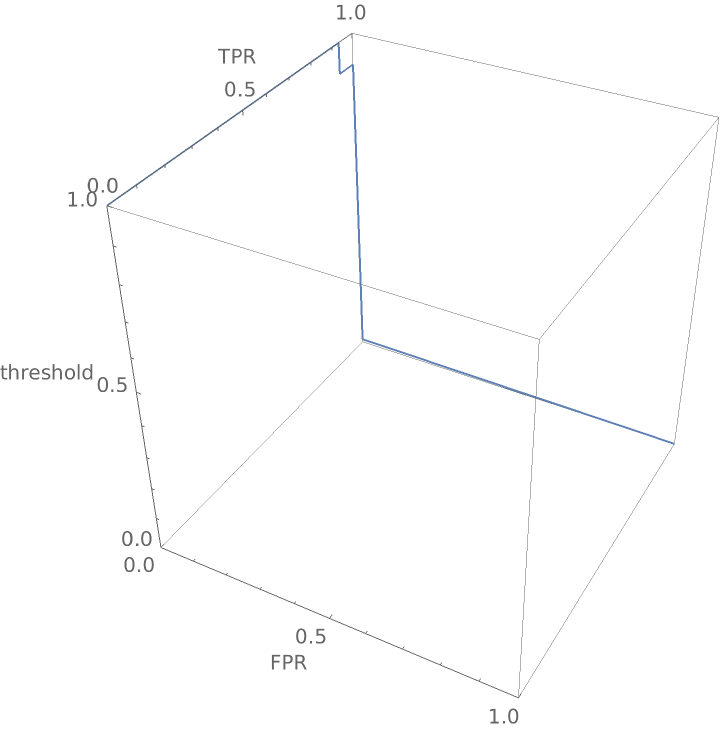Create a confusion matrix trajectory function as before, but let "virginica" be designated as the positive class:

 In:=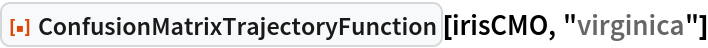Out=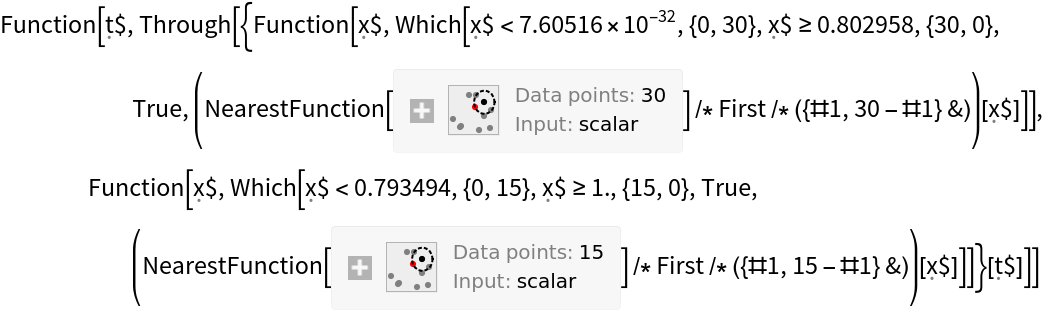Get the confusion matrix if the threshold is 0.62:

 In:=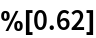Out=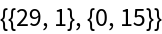### Scope (2)

One does not need a ClassifierMeasurementsObject to create the requisite function; the function will work with an Association that has the requisite key-value pairs as shown in the following example:

 In:=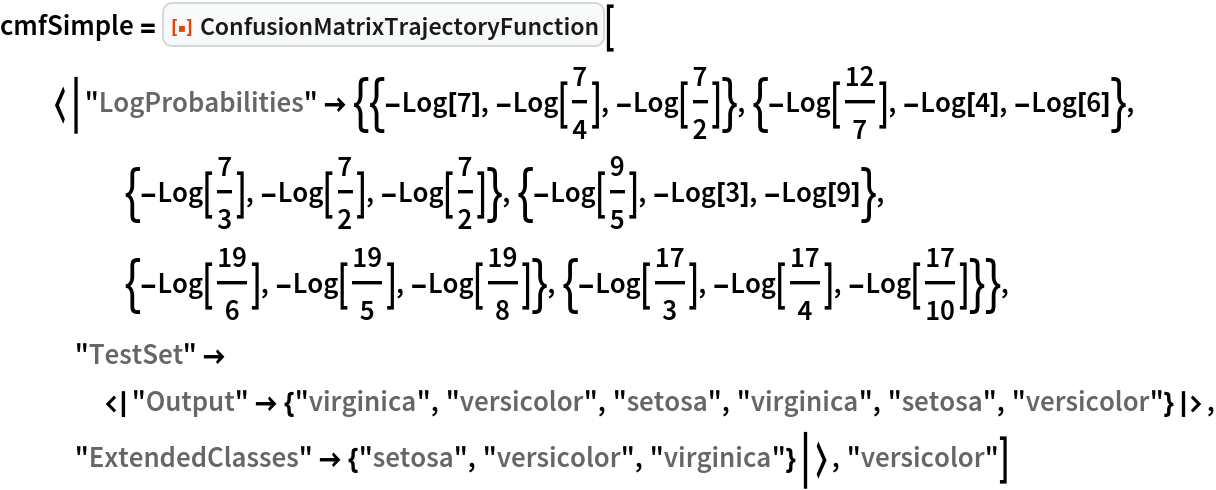Out=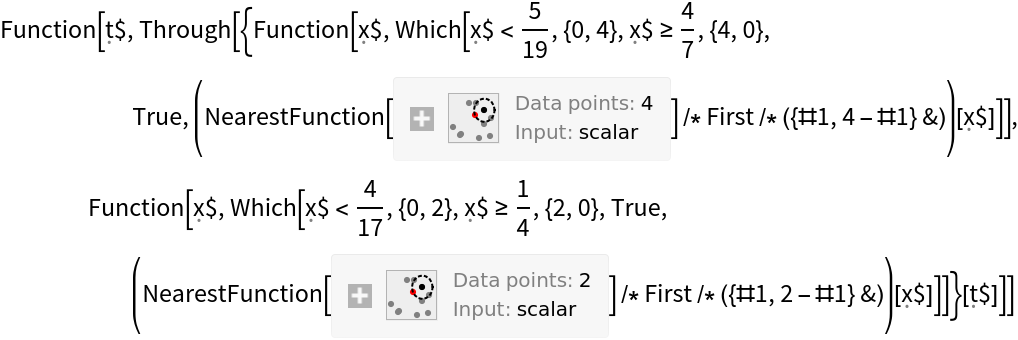One can use the function on a neural network that has been converted to a classifier. Create a trained network following the method set forth here:

 In:=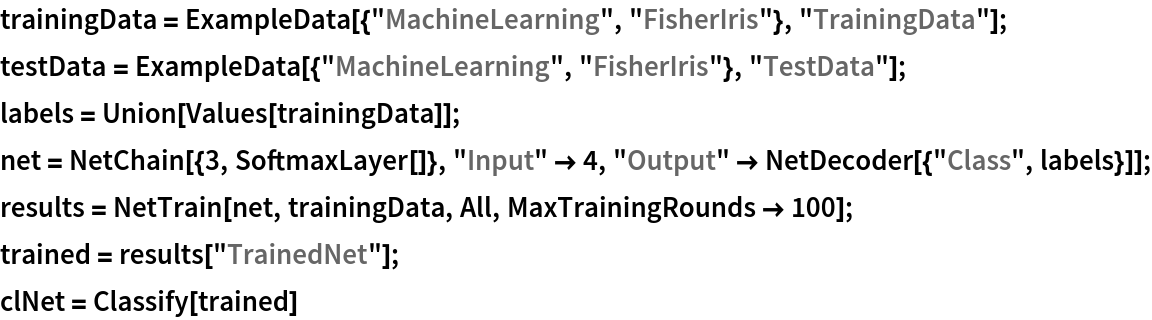Out=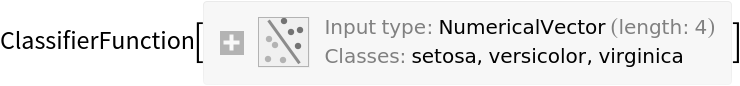Create a ClassifierMeasurementsObject using testData:

 In:=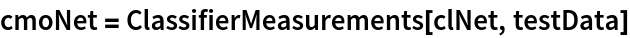Out=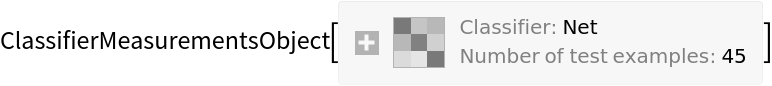Create the confusion matrix trajectory function with "versicolor" as the positive class:

 In:=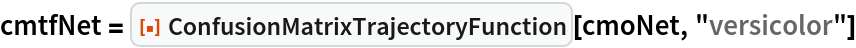Out=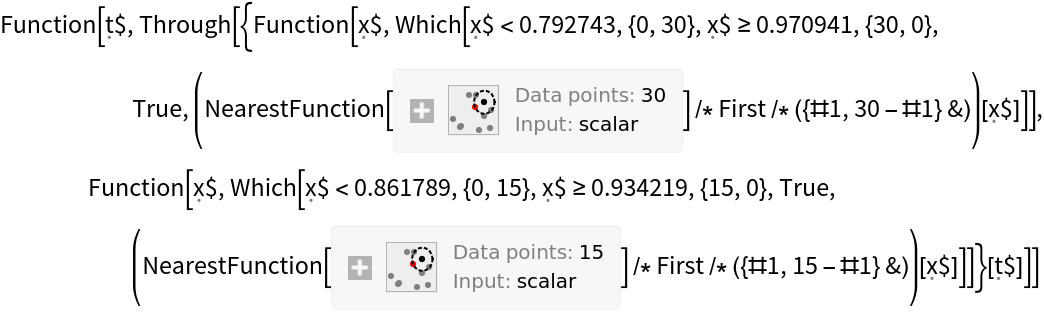Show the three-dimensional ROC (receiver operating characteristic) curve for the network:

 In:=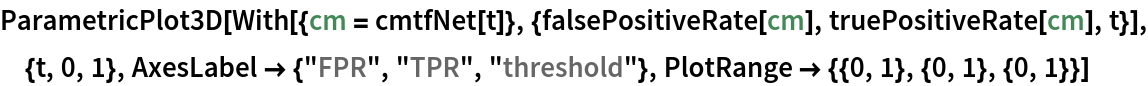Out=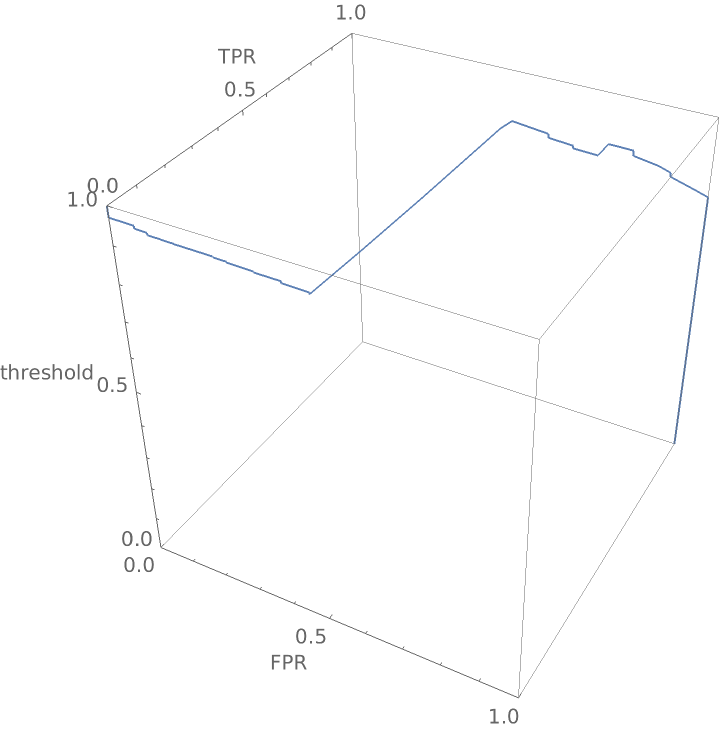### Applications (2)

Create functions that compute the false positive rate and true positive rate from a confusion matrix:

 In:=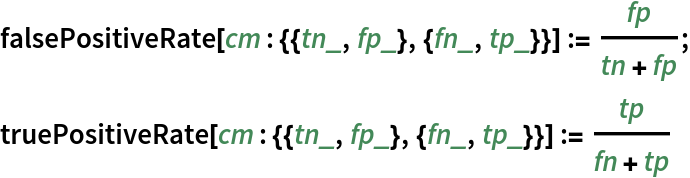Construct a three-dimensional receiver operating curve that shows the false positive rate and true positive rate for "good" wine as a function of the threshold probability required before the classifier calls a wine "good":

 In:=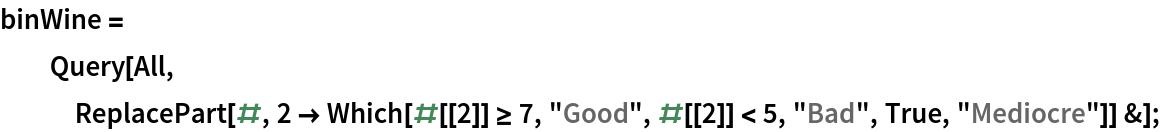In:=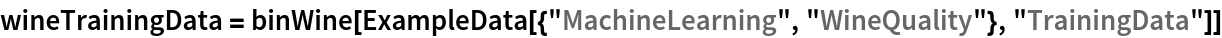Out=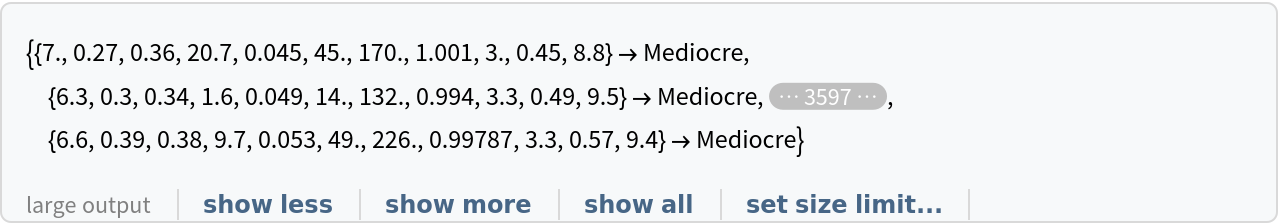In:=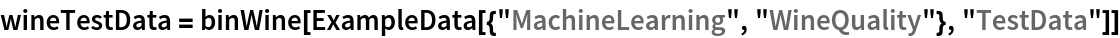Out=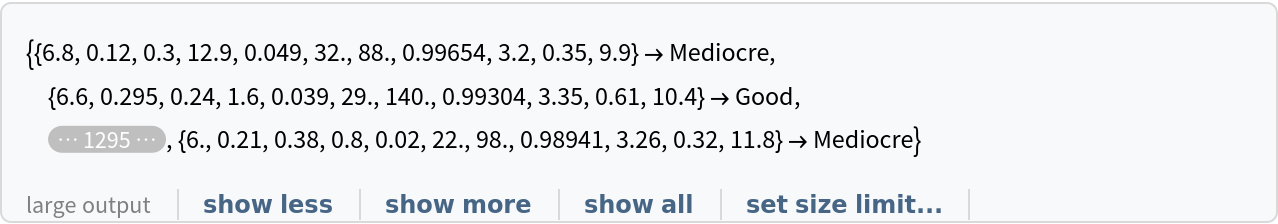In:=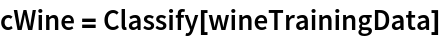Out=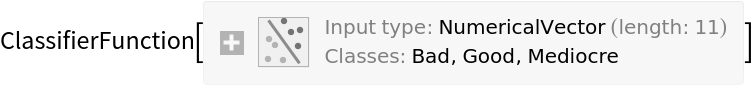Construct a ClassifierMeasurementsObject from the classifier and the test set:

 In:=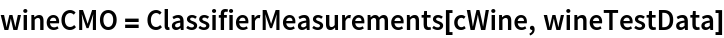Out=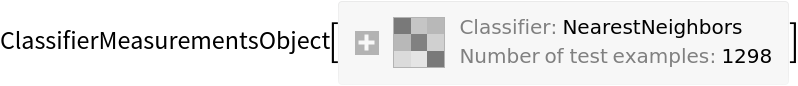Create the confusion matrix function:

 In:=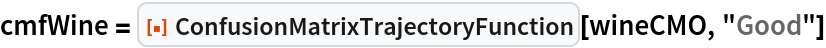Out=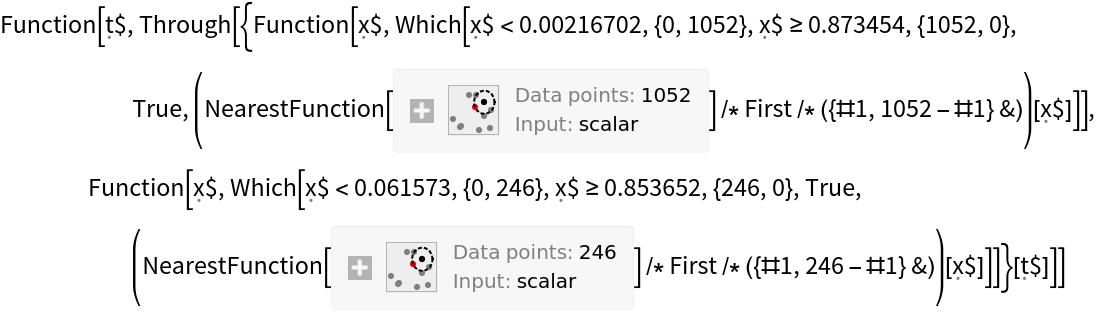Create a three-dimensional plot:

 In:=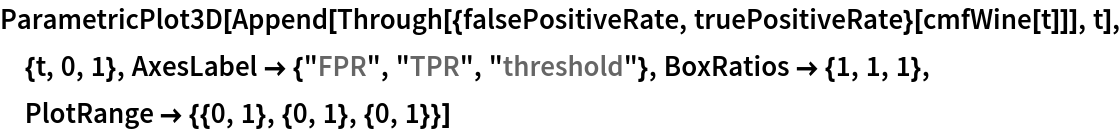Out=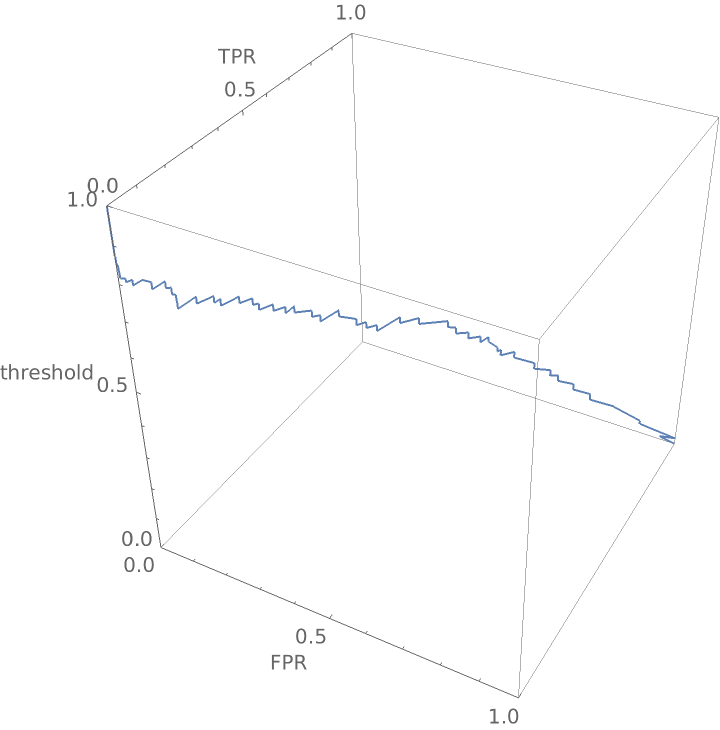View the graphic from the top as a traditional receiver operating curve:

 In:=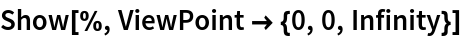Out=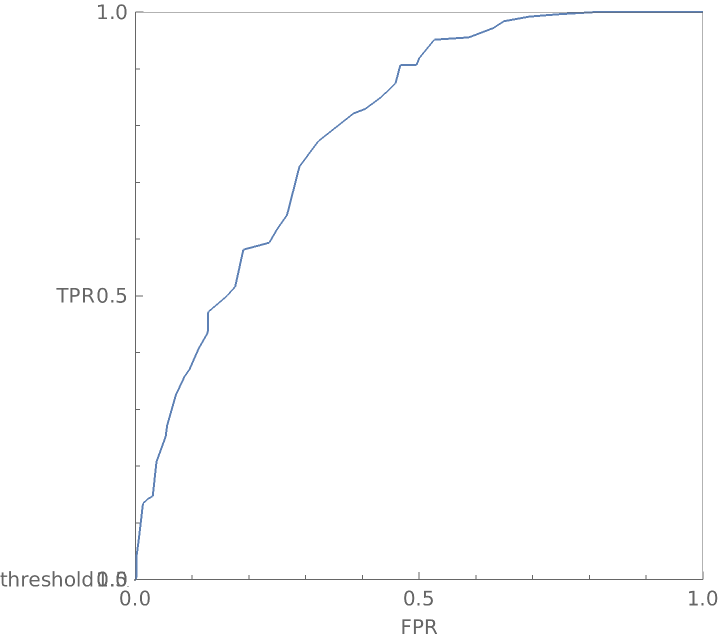The function works well in conjunction with the CrossValidateModel resource function and permits one to smooth the confusion matrix trajectory. Start this process by creating a query that will convert continuous wine quality values into discrete bins of Good, Bad and Mediocre:

 In:=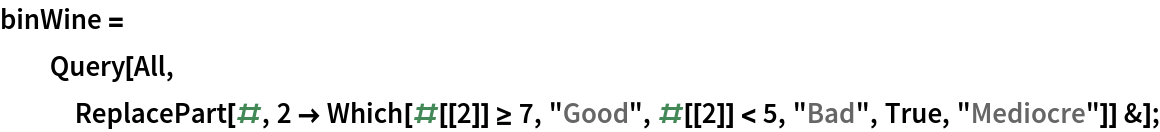Create wine data suitable for Classify:

 In:=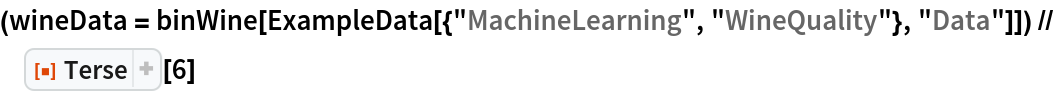Out=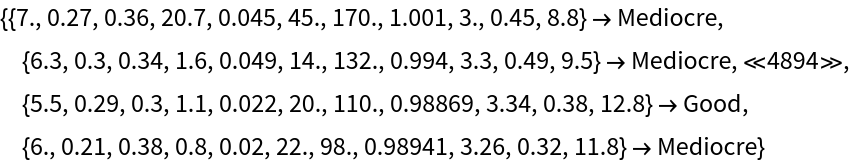Run the CrossValidateModel resource function to create five pairs of ClassifierFunction and ClassifierMeasurementsObject for each of the five folds:

 In:=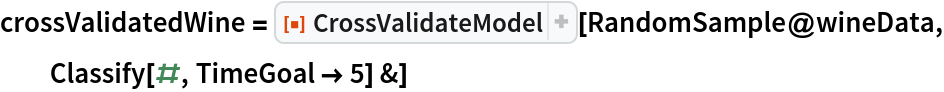Out=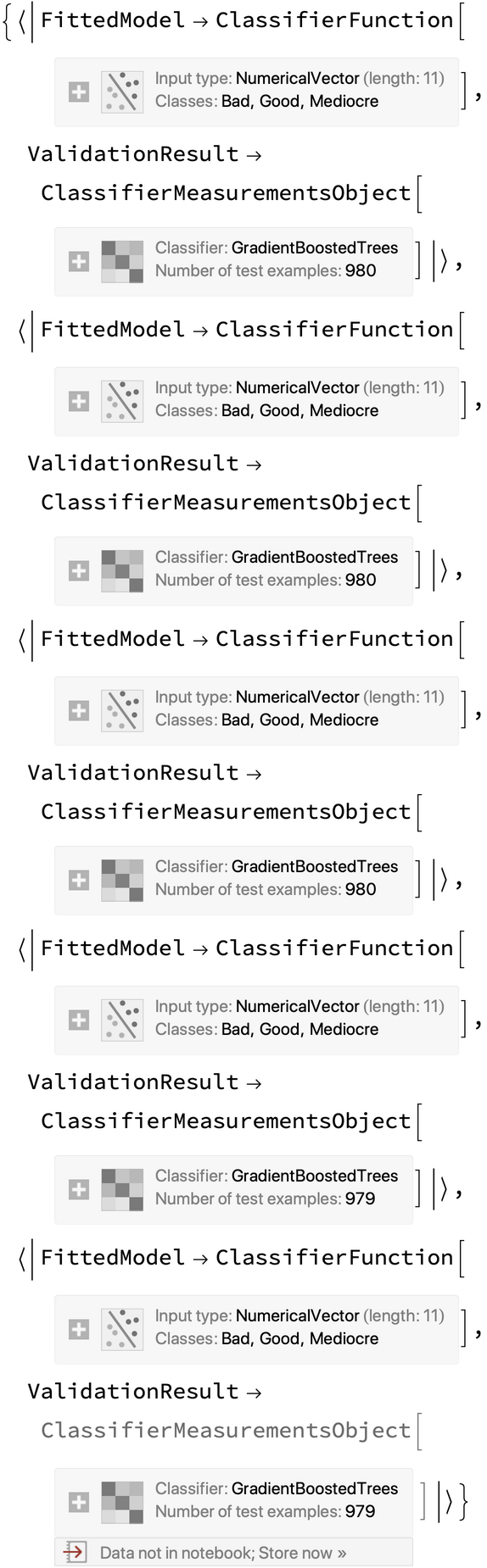Create confusion matrix trajectory functions for each of the five folds:

 In:=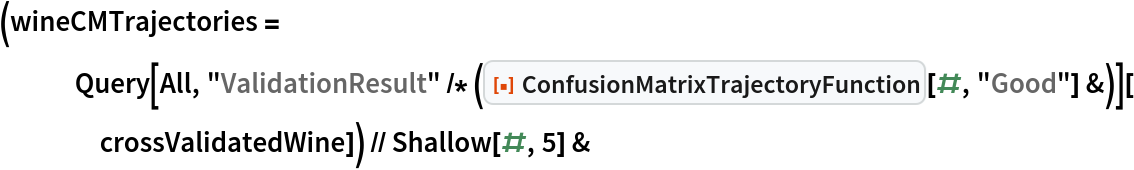Out=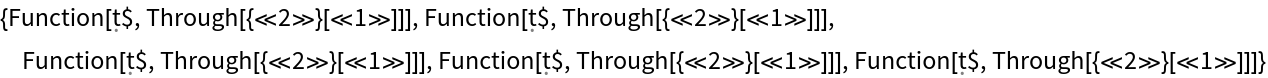Plot the accuracy of the classifier over the threshold levels for each of the folds:

 In:=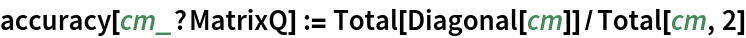In:=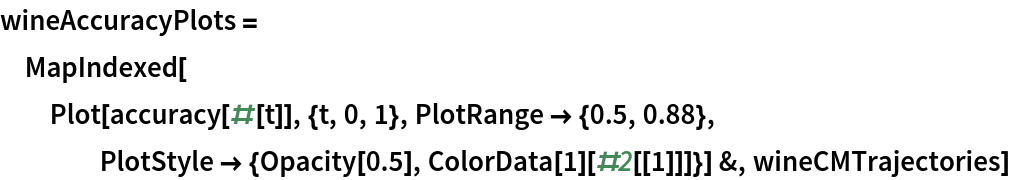Out=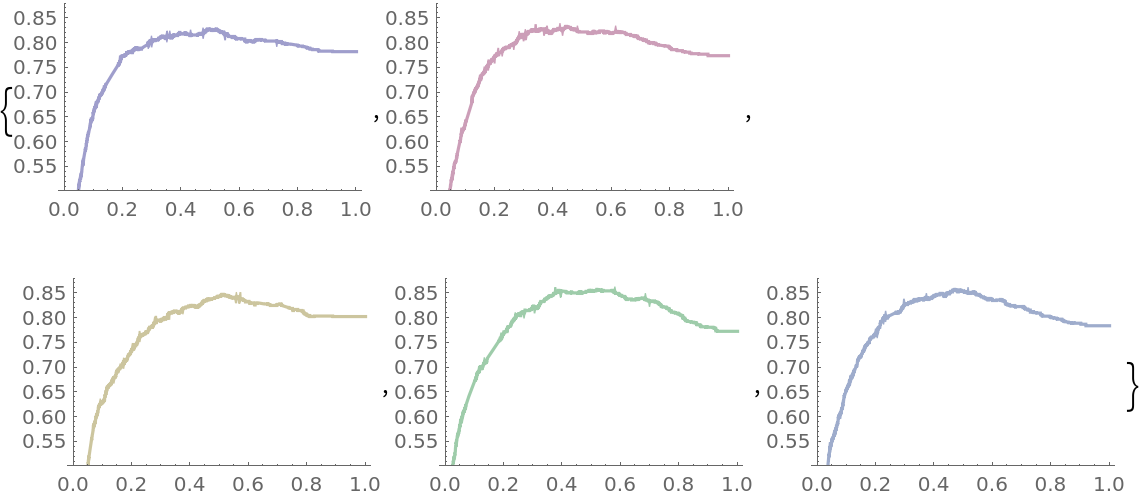Create a composite view of the five accuracy plots:

 In:=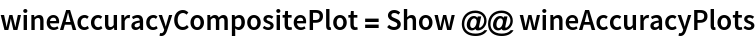Out=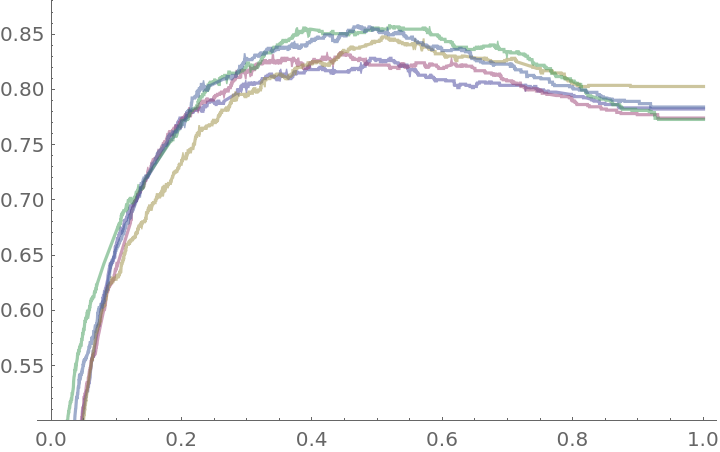Now create a function that computes confusion matrices for all five folds:

 In:=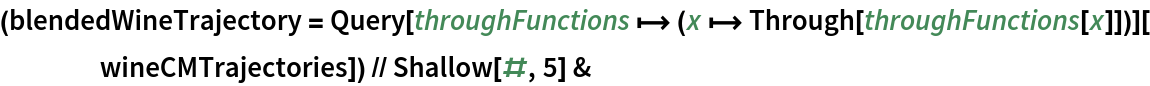Out=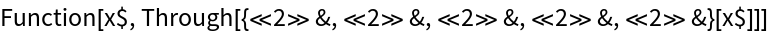Plot the blended accuracy curve:

 In:=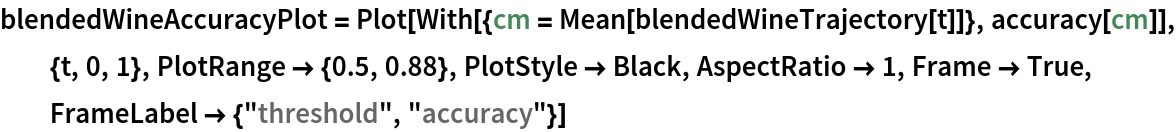Out=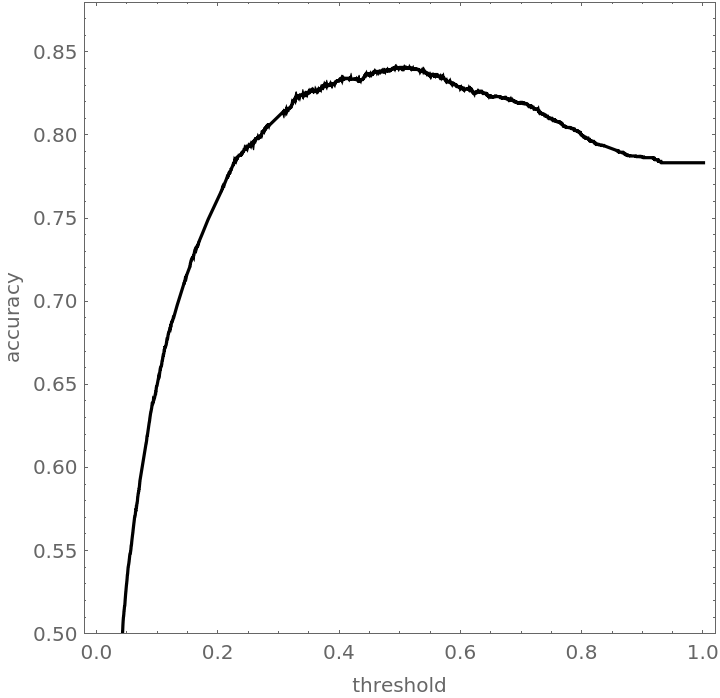Show the blended accuracy plot and the components creating it:

 In:=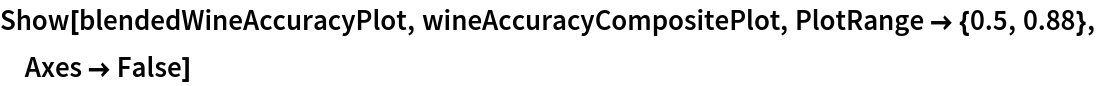Out=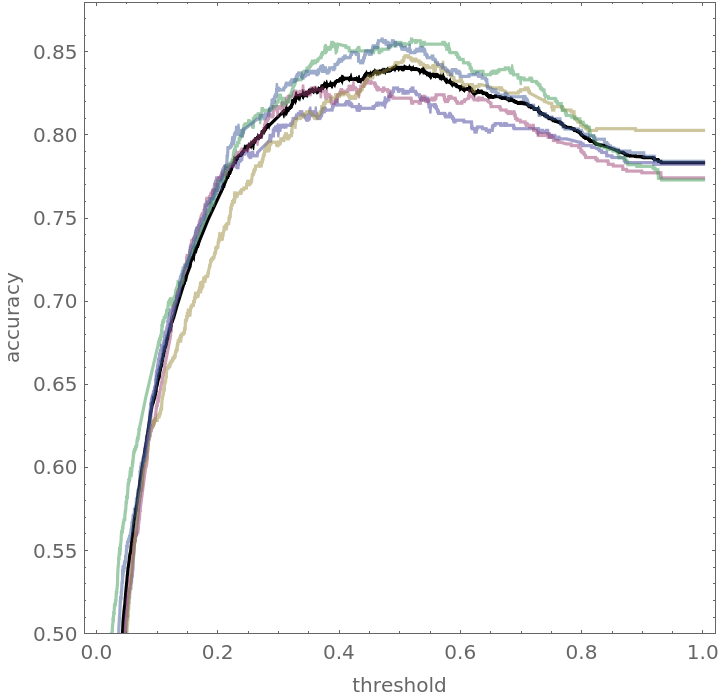### Neat Examples (4)

Compare how a classifier performs on wines with low pH versus wines with high pH:

 In:=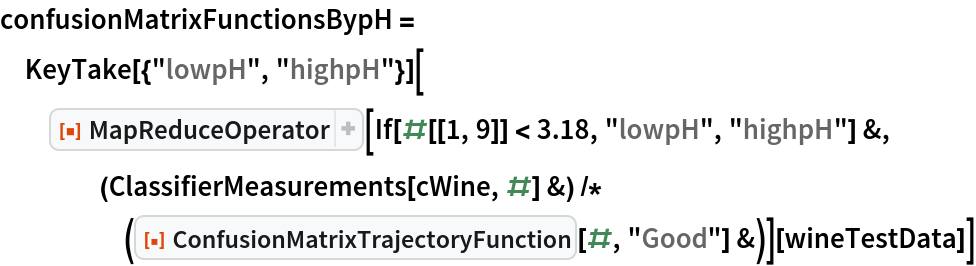Out=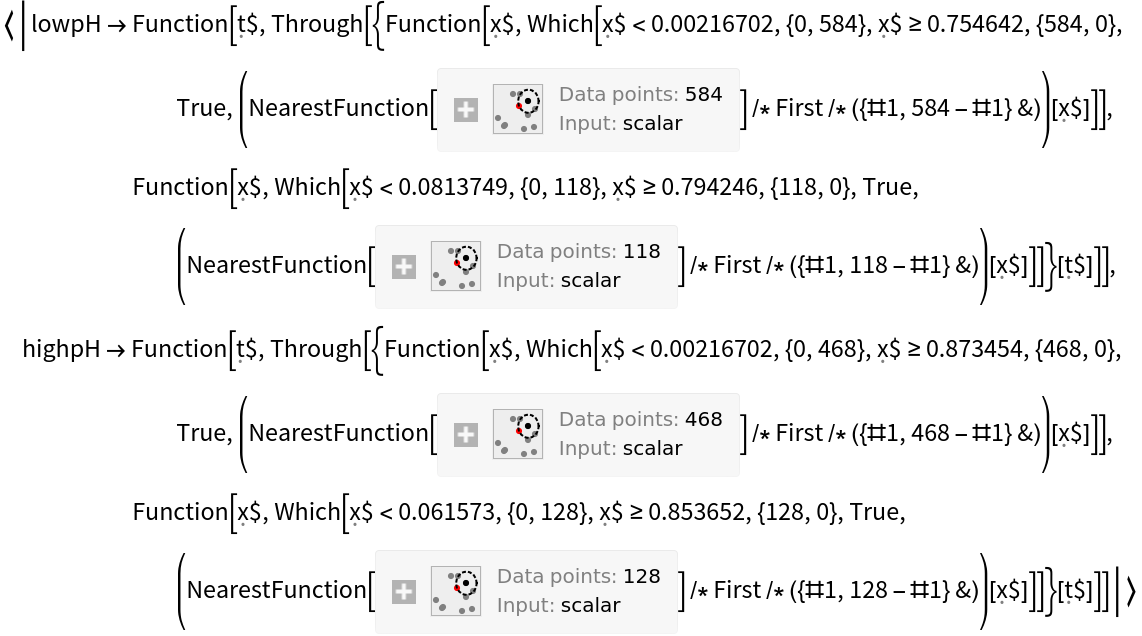Show the three-dimensional ROC curves and draw points where the threshold is 0.5:

 In:=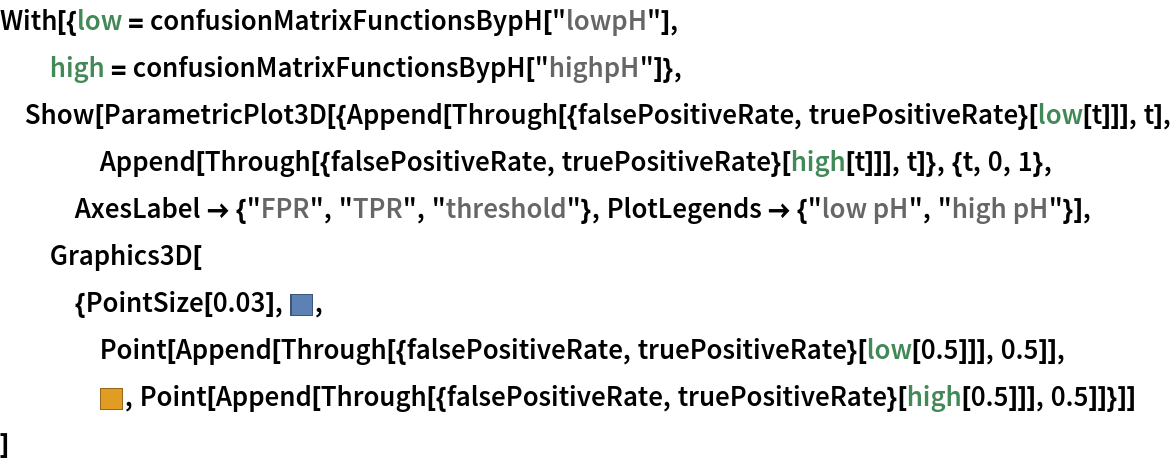Out=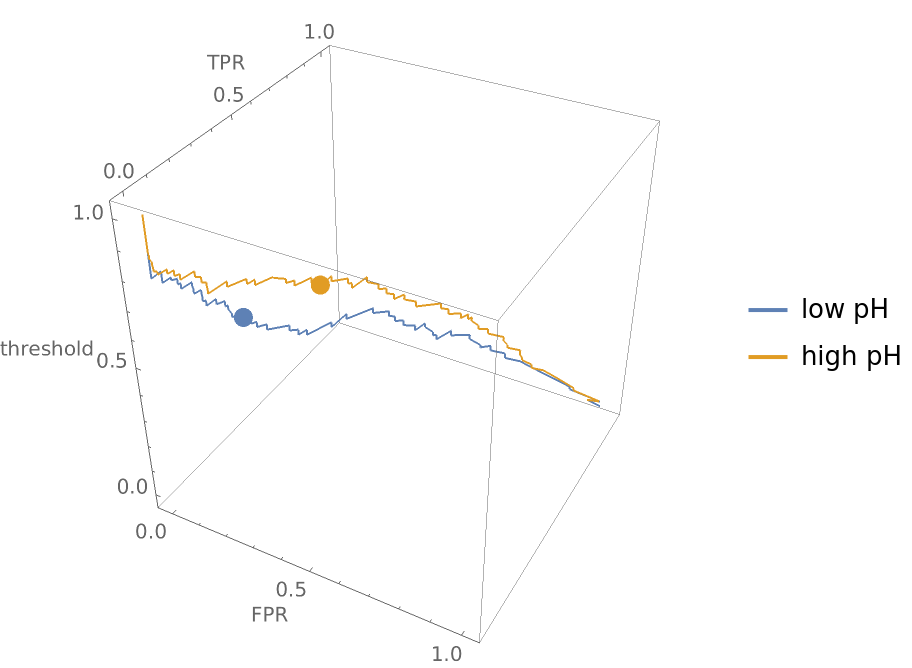Show the graphic from the top and notice that even though the ROC curves look roughly the same in two dimensions, the false positive and true positive rates for the two different pH groupings of wine differ significantly at a threshold of 0.5:

 In:=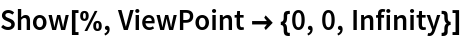Out=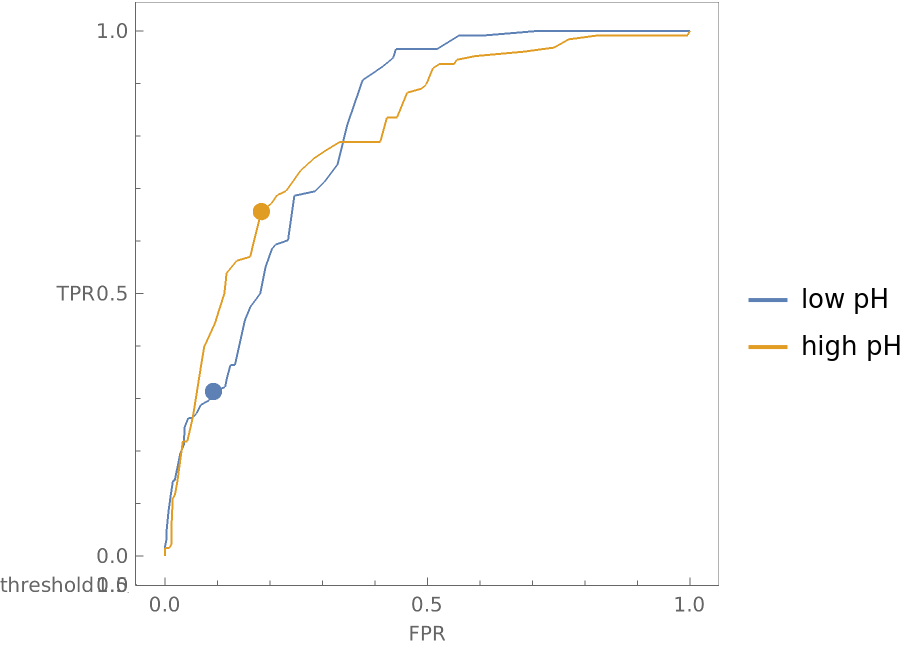Create the same plot but make the hue of each plot line depend on the accuracy of the classifier at that threshold:

 In:=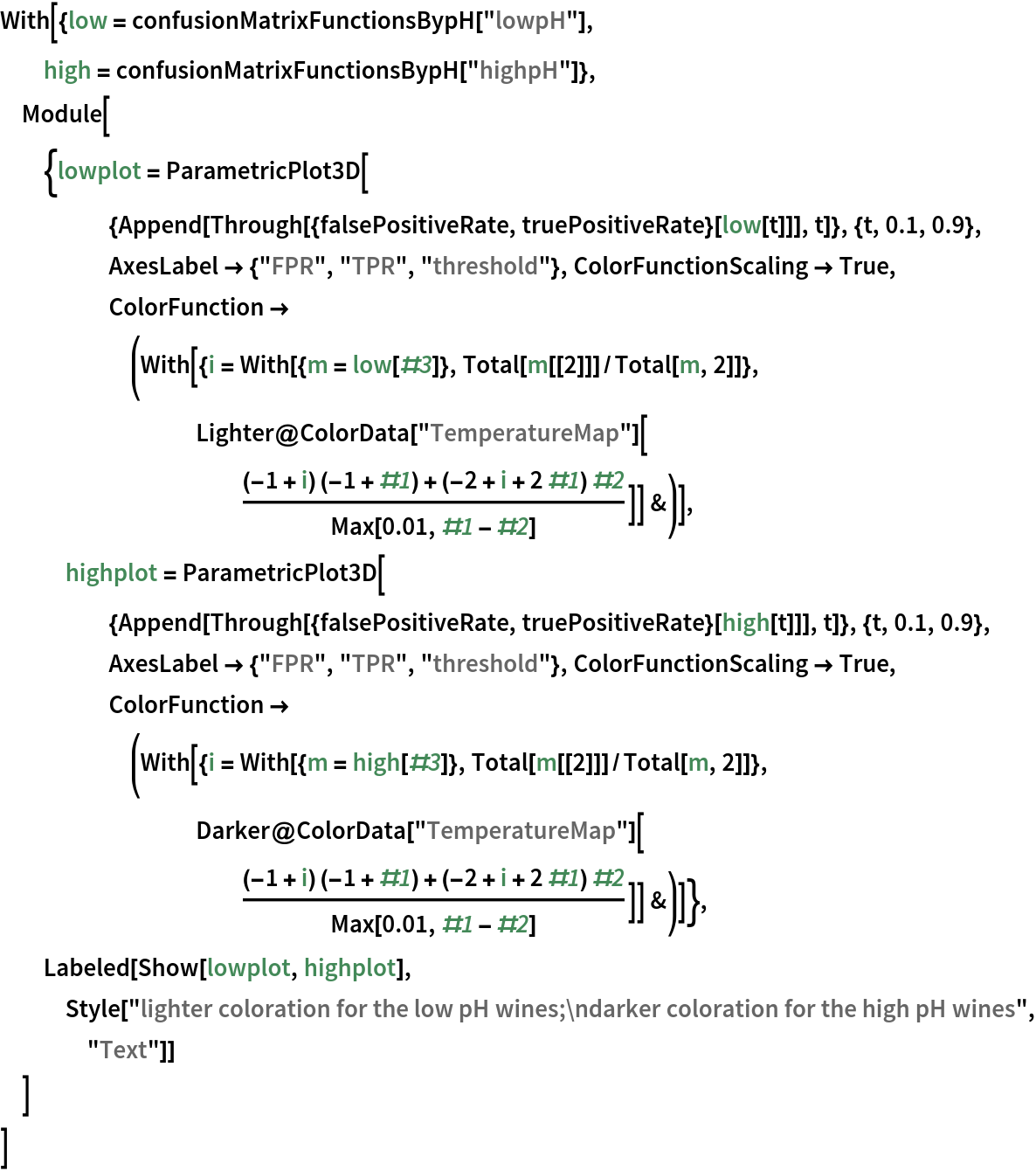Out=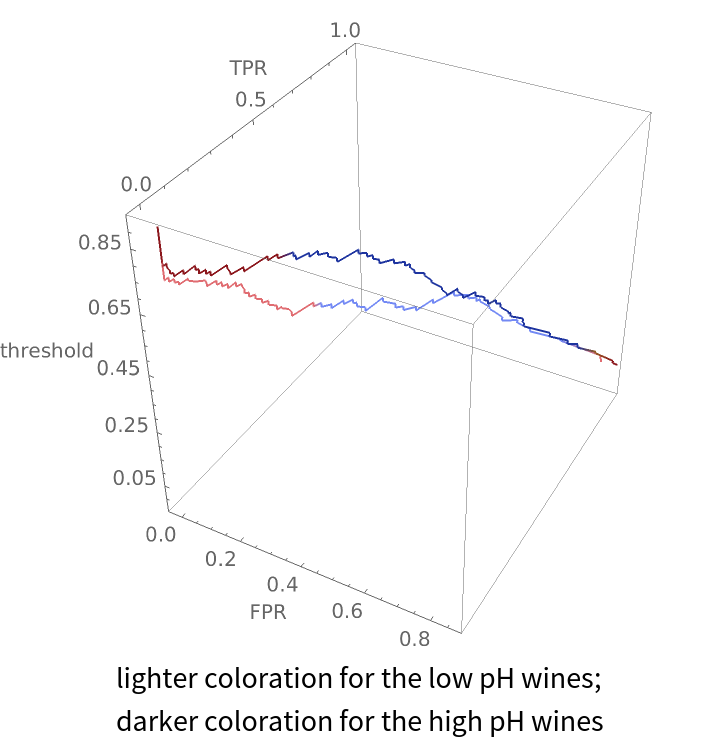Seth J. Chandler

## Version History

• 1.0.0 – 27 October 2020

## Author Notes

The motivation for this function is efficient pursuit of the issue shown in Neat Examples, which is understanding and examining the difference in the performance of a classifier on various subgroups of a dataset. It is thus useful in the area of "Fair Machine Learning" or algorithmic bias.